# Projective limit

(diff) ← Older revision | Latest revision (diff) | Newer revision → (diff)

inverse limit

A construction that arose originally in set theory and topology, and then found numerous applications in many areas of mathematics. A common example of a projective limit is that of a family of mathematical structures of the same type indexed by the elements of a pre-ordered set. Letbe a set endowed with a pre-order relation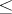, and suppose that with each element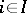a set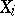is associated and with each pair,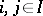, in which, a mappingis associated, where the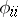,, are identity mappings and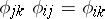for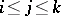. A setis called a projective limit of the family of setsand mappingsif the following conditions are satisfied: a) there exists a family of mappings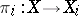such thatfor any pair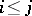; b) for any family of mappings,, from an arbitrary set, for which the equalitieshold for, there exists a unique mappingsuch that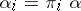for every. The projective limit can be described explicitly as follows. One considers the direct product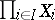and selects in it the set of all functions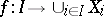for which the equalitieshold for. This subset is the projective limit of the family. If all theare equipped with an additional structure of the same type and the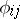preserve it, then the same structure is induced in the projective limit. Therefore it is possible to speak of projective limits of groups, modules, topological spaces, etc.

A natural generalization of this concept of projective limit is that of the projective limit of a functor. Let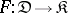be a functor from a small categoryto an arbitrary category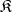. An object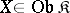together with morphisms,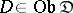, is called a projective limit (inverse limit, or simply limit) of the functorif the following conditions are satisfied:)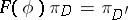for any morphism; and) for any family of morphisms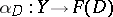satisfying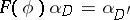for all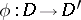there exists a unique morphism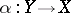such that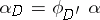for all. Notation: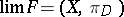.

### Examples of projective limits.

1) Letbe a discrete category. Then for an arbitrary functor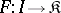the projective limit of the functorcoincides with the product of the family of objects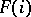,(cf. Product of a family of objects in a category).

2) Let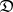be a category with two objects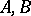and two non-identity morphisms. Then the limit of a functoris the equalizer of the pair of morphisms(cf. Kernel of a morphism in a category).

If a category has products of arbitrary small families of objects and equalizers of pairs of morphisms, then it has limits for all functors defined on small categories.